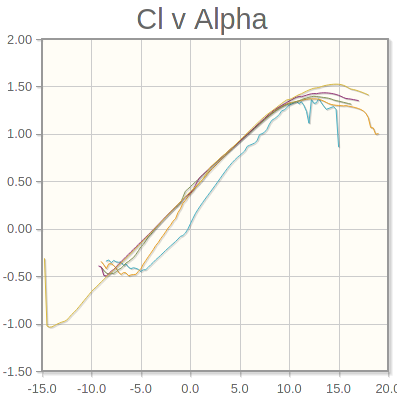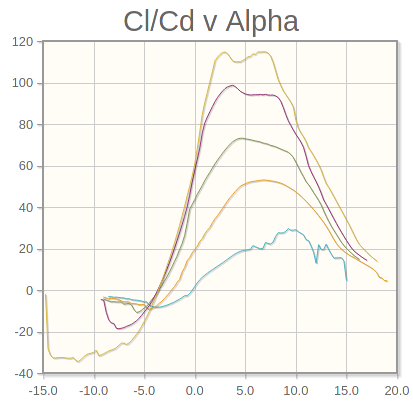# Iteration 2: Weight and Airfoil#

from unyt import km, m, mm, g, kg, hr, s, degree, radian
import numpy as np
from math import pi as π

ρ = 1.225*kg/m**3 # air density
μinf = 1.81e-5*kg/(m*s) # viscosity of air


Goals:

• Decrease aspect ratio

## Initial Design#

b = 2850*mm # wingspan
c = 230*mm # chord

S = b*c # wing area
AR = b**2/S

AR

unyt_quantity(12.39130435, '(dimensionless)')


Better, although I may have overdone it

## Weight Estimate#

W = 3700*g # weight


## Airfoil Selection#

V = 45*km/hr # cruise speed


Randomly selected, see https://rcmodelhub.com/how-fast-do-rc-planes-go/#0-typical-speeds-of-popular-rc-planes-

CL = (2*W)/(ρ*V**2*S)
CL.to('s**2/m')

unyt_quantity(0.05897975, 's**2/m')

Re = (ρ*V*c)/μinf
round(Re.to_value(), -3)

195000.0


As per: http://airfoiltools.com/airfoil/details?airfoil=clarky-il
Clark Y chosen because:

• No need for inverted flight

• Long term goal is efficiency

• Relatively easy foam manufacturingFrom bottom left up: Blue = 50,000 Orange = 100,000 Green = 200,000

# rough estimations
α0 = -3.75*degree
Clα = 1.05
e = 0.8

CLα = Clα/(1+(Clα/(π*e*AR)))
CLα

unyt_quantity(1.01575315, '(dimensionless)')

CL_unitless = CL.to_value('s**2/m')
CL_unitless

0.05897974750540949

α = α0+((CL_unitless/CLα)*radian)
α

unyt_quantity(-0.42311827, 'degree')